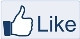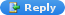# IMAGE PROCESSING

" Two roads diverged in a wood, and I,
I took the one less traveled by,
And that has made all the difference "-Robert Frost

### Principal Component Analysis

Principal component analysis is a statistical technique that is used in finding patterns and reducing the dimensions of multi-dimensional data. There is an excellent tutorial by Lindsay I Smith on this topic so I will be focusing more on the application part in this post.

EXAMPLE:

MATLAB CODE:
clear all
clc

figure(1),subplot(121),imshow(I);title('INPUT IMAGE');

A = double(I);

%MEAN OF EACH COLUMN IN THE IMAGE
meanCols = mean(A,1);

%SUBTRACT THE MEAN VALUE OF EACH COLUMN
for k = 1:size(A,1)
A(k,:) = A(k,:)-meanCols;
end

%COMPUTE COVARIANCE MATRIX
covmat = cov(A);
%OBTAIN EIGEN VALUES
[coeff,D] = eig(covmat);
coeff = fliplr(coeff);
figure(2),imagesc(coeff);colormap(jet);
FV = coeff(:,1:50)'; %PRINCIPAL COMPONENT
Res = FV*A';

%RECONSTRUCTION
Org = (FV'*Res)';

%ADD THE MEAN VALUES OF THE COLUMN
Output = zeros(size(A));
for k = 1:size(A,1)
Output(k,:) = Org(k,:)+meanCols;
end

Output = uint8(Output);
figure(1),subplot(122),imshow(Output);title('RESTORED IMAGE');

EXAMPLE 2:

Let’s consider the LANDSAT-8 dataset. I have chosen the following bands.
Band 2– BLUE,
Band 3 – Green,
Band 4 – Red,
Band 5 – NIR
Band 6 - SWIR-1
Band 7 – SWIR-2

MATLAB CODE:
clear all

% READ ALL THE TIF IMAGE NAMES
fnames = dir('*.TIF');
dim1=400;
dim2=950;
C=[];
inc=1;

%CREATE A VOXEL OF IMAGES
for k = [4,5,6,7,8,9];

A = A(1901:2300,2251:3300);
inc=inc+1;
C =[C,A(:)];
end

C1 = double(C);
%SUBTRACT THE MEAN
meanval = zeros([1,size(C,2)]);
for k = 1:size(C,2)
meanval(1,k) = mean(squeeze(C(:,k)));
C1(:,k) = C1(:,k)- meanval(1,k);
end

%PERFORM PCA ANALYSIS
coeff = pca(C1,'Algorithm','eig');
figure,imagesc(coeff);colormap(jet);
FV=coeff(:,[1:3])'; %PRINCIPAL COMPONENTS

Res = FV*C1';

%RECONSTRUCTION
Org = (FV'*Res)';

%2-Blue, 3-Green 4-Red, 5-NIR, 6 and 7 SWIR-1 and 2
%NATURAL COLOR
R1 = Org(:,3)+mean(C(:,3));
G1 = Org(:,2)+mean(C(:,2));
B1 = Org(:,1)+mean(C(:,1));

R1= reshape(R1,[size(A,1) size(A,2)]);
G1= reshape(G1,[size(A,1) size(A,2)]);
B1= reshape(B1,[size(A,1) size(A,2)]);

%ENHANCE THE CONTRAST
R1 = BCET16(0,65535,20000,R1);
G1 = BCET16(0,65535,20000,G1);
B1 = BCET16(0,65535,20000,B1);

Output = zeros([size(A,1) size(A,2) 3]);
Output(:,:,1)=R1;
Output(:,:,2)=G1;
Output(:,:,3)=B1;

figure(8),imagesc(uint16(Output));title('Natural Color');

%6-SWIR-2,4-NIR,2-GREEN
R1 = Org(:,6)+mean(C(:,6));
G1 = Org(:,4)+mean(C(:,4));
B1 = Org(:,2)+mean(C(:,2));

R1= reshape(R1,[size(A,1) size(A,2)]);
G1= reshape(G1,[size(A,1) size(A,2)]);
B1= reshape(B1,[size(A,1) size(A,2)]);

%ENHANCE THE CONTRAST
R1 = BCET16(0,65535,25000,R1);
G1 = BCET16(0,65535,25000,G1);
B1 = BCET16(0,65535,25000,B1);

Output1 = zeros([size(A,1) size(A,2) 3]);
Output1(:,:,1)=R1;
Output1(:,:,2)=G1;
Output1(:,:,3)=B1;
figure(4),imagesc(uint16(Output1));title('Vegetation and Water');

%SWIR-1, SWIR-2,Red
%Urban
R1 = Org(:,6)+mean(C(:,6));
G1 = Org(:,5)+mean(C(:,5));
B1 = Org(:,3)+mean(C(:,3));

R1= reshape(R1,[size(A,1) size(A,2)]);
G1= reshape(G1,[size(A,1) size(A,2)]);
B1= reshape(B1,[size(A,1) size(A,2)]);

%ENHANCE THE CONTRAST
R1 = BCET16(0,65535,25000,R1);
G1 = BCET16(0,65535,25000,G1);
B1 = BCET16(0,65535,25000,B1);

Output2 = zeros([size(A,1) size(A,2) 3]);
Output2(:,:,1)=R1;
Output2(:,:,2)=G1;
Output2(:,:,3)=B1;
figure(5),imagesc(uint16(Output2));title('Urban Environment');

%SWIR-1,NIR,RED(4,3,2)
%Color IR
R1 = Org(:,4)+mean(C(:,4));
G1 = Org(:,3)+mean(C(:,3));
B1 = Org(:,2)+mean(C(:,2));

R1= reshape(R1,[size(A,1) size(A,2)]);
G1= reshape(G1,[size(A,1) size(A,2)]);
B1= reshape(B1,[size(A,1) size(A,2)]);

%ENHANCE THE CONTRAST
R1 = BCET16(0,65535,25000,R1);
G1 = BCET16(0,65535,25000,G1);
B1 = BCET16(0,65535,25000,B1);

Output3 = zeros([size(A,1) size(A,2) 3]);
Output3(:,:,1)=R1;
Output3(:,:,2)=G1;
Output3(:,:,3)=B1;
figure(6),imagesc(uint16(Output3));title('Color IR');

EXPLANATION:

The total number of bands chosen is 6. PC1, PC2 and PC3 are used to reconstruct the 6 bands. PC1 is the largest contributor. By combining different bands together we obtain natural color, urban environment, vegetation and color IR.

NOTE: The dataset is obtained from https://landsatlook.usgs.gov/

The function BCET16 is used to enhance the contrast. Save the below function as BCET16.m and make sure the m file is available in the current working directory.

function y=BCET16(Gmin,Gmax,Gmean,x)
%BALANCE CONTRAST ENHANCEMENT TECHNIQUE
x    = double(x); % INPUT IMAGE
Lmin = min(x(:)); % MINIMUM OF INPUT IMAGE
Lmax = max(x(:)); % MAXIMUM OF INPUT IMAGE
Lmean = mean(x(:)); %MEAN OF INPUT IMAGE
LMssum = mean(x(:).^2); %MEAN SQUARE SUM OF INPUT IMAGE

bnum = Lmax.^2*(Gmean-Gmin) - LMssum*(Gmax-Gmin) + Lmin.^2*(Gmax-Gmean);
bden = 2*(Lmax*(Gmean-Gmin)-Lmean*(Gmax-Gmin)+Lmin*(Gmax-Gmean));

b = bnum/bden;

a = (Gmax-Gmin)/((Lmax-Lmin)*(Lmax+Lmin-2*b));

c = Gmin - a*(Lmin-b).^2;

y = a*(x-b).^2+c; %PARABOLIC FUNCTION
y = uint16(y);
endLike "IMAGE PROCESSING" pageAnonymous said...Hello sir, I have a number of satellite images and I want to reduce their dimensions using two methods (factor analysis and multidimensional scaling), do you have a code for these two methods please, can you help me please, I am very need for any help and I would be thankful to youAnonymous said...Hello sir, I have a number of satellite images and I want to reduce their dimensions using two methods (factor analysis and multidimensional scaling), do you have a code for these two methods or any of them please, can you help me please, I am very need for any help and I would be thankful to you

Unknown said...Dual Polynomial Thresholding For Transform
Denoising In Application To Local Pixel Grouping
Method
Plz send this code

Unknown said...sir i have hyperion datasets i want to do the spectral unmixing can you please send me the code

Unknown said...sir i have hyperion data sets which is hyperspectral image i want to do spectral unmixing can you send me the code